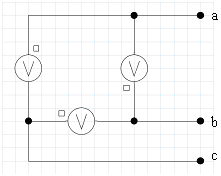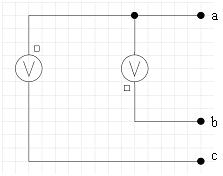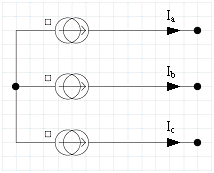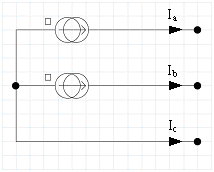# Specific cases for three-phase sources

## Introduction

The analysis methods used by Flux PEEC for taking into account the equations corresponding to the electrical sources (voltage and current) does not allow the representation of the following two types of ideal balanced three-phase sources by their real schematic:

• D-connected three-phase voltage source
• Y-connected three-phase current source

Their real schematic will in fact lead to computational errors, which can be easily overcome by representing only two single-phase sources, as indicated here below.

## Δ-connected three-phase voltage source

The real schematic of an ideal balanced D-connected three-phase voltage source should be composed of three single-phase sources of equal magnitude and with 120° phase between them (see left figure). Within Flux PEEC – Supplied Conductors (AC or DC) applications, to overcome computational problems, it is necessary to remove one voltage source (see right figure below). This schematic will impose the correct potentials at nodes a, b and c of the circuit.

• real schematic: do not use in Flux PEEC• correct schematic: use in Flux PEEC## Y–connected three-phase current source

The real schematic of an ideal balanced Y–connected three-phase current source should be composed of three single-phase sources of equal magnitude and with 120° phase between them (see left figure). Within Flux PEEC – Supplied Conductors (AC or DC) applications, to overcome computational problems, it is necessary to remove one current source (see right figure below). This schematic will impose in the circuit the correct currents Ia, Ib and Ic.

• real schematic: do not use in Flux PEEC• correct schematic: use in Flux PEEC